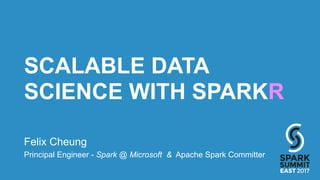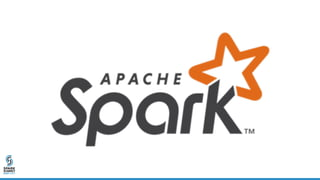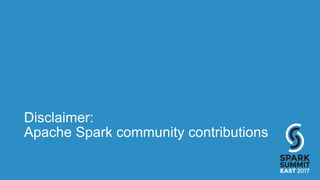Successfully reported this slideshow.

# Scalable Data Science with SparkR: Spark Summit East talk by Felix Cheung×

# Scalable Data Science with SparkR: Spark Summit East talk by Felix Cheung

R is a very popular platform for Data Science. Apache Spark is a highly scalable data platform. How could we have the best of both worlds? How could a Data Scientist leverage the rich 9000+ packages on CRAN, and integrate Spark into their existing Data Science toolset?
In this talk we will walkthrough many examples how several new features in Apache Spark 2.x will enable this. We will also look at exciting changes in and coming next in Apache Spark 2.x releases.

R is a very popular platform for Data Science. Apache Spark is a highly scalable data platform. How could we have the best of both worlds? How could a Data Scientist leverage the rich 9000+ packages on CRAN, and integrate Spark into their existing Data Science toolset?
In this talk we will walkthrough many examples how several new features in Apache Spark 2.x will enable this. We will also look at exciting changes in and coming next in Apache Spark 2.x releases.

### Scalable Data Science with SparkR: Spark Summit East talk by Felix Cheung

1. 1. SCALABLE DATA SCIENCE WITH SPARKR Felix Cheung Principal Engineer - Spark @ Microsoft & Apache Spark Committer
2. 2. Disclaimer: Apache Spark community contributions
3. 3. Spark in 5 seconds • General-purpose cluster computing system • Spark SQL + DataFrame/Dataset + data sources • Streaming/Structured Streaming • ML • GraphX
4. 4. R • A programming language for statistical computing and graphics • S – 1975 • S4 - advanced object-oriented features • R – 1993 • S + lexical scoping • Interpreted • Matrix arithmetic • Comprehensive R Archive Network (CRAN) – 10k+ packages
5. 5. SparkR • R language APIs for Spark and Spark SQL • Exposes Spark functionality in an R-friendly DataFrame APIs • Runs as its own REPL sparkR • or as a R package loaded in IDEs like RStudio   library(SparkR)  sparkR.session()
6. 6. Architecture • Native R classes and methods • RBackend • Scala “helper” methods (ML pipeline etc.) www.slideshare.net/SparkSummit/07-venkataraman-sun
7. 7. Advantages • JVM processing, full access to DAG capabilities and Catalyst optimizer, predicate pushdown, code generation, etc. databricks.com/blog/2015/06/09/announcing-sparkr-r-on-spark.html
8. 8. Features - What’s new in SparkR • SQL • Data source (JSON, csv, PostgreSQL, libsvm) • SparkSession & default session (streamlined parameter) as.DataFrame(iris) • Catalog (external data table management) • Spark packages, spark.addFiles() • ML • R-native UDF • Cluster support (YARN, mesos, standalone)
9. 9. SparkR for Data Science
10. 10. Decisions, decisions? Distributed? Native R UDF Spark.ml YesNo
11. 11. Spark ML Pipeline • Pre-processing, feature extraction, model fitting, validation stages • Transformer • Estimator • Cross-validation/hyperparameter tuning Tokenizer HashTF Logistic Regression
12. 12. SparkR API for ML Pipeline spark.lda(  data = text, k = 20, maxIter = 25, optimizer = "em") RegexTokenizer StopWordsRemover CountVectorizer R JVM LDA Single-entrypoint  R API builds JVM ML Pipeline
13. 13. Model Operations • summary - print a summary of the fitted model • predict - make predictions on new data • write.ml/read.ml - save/load fitted models (slight layout difference: pipeline model plus R metadata)
14. 14. Spark.ml in SparkR 2.0.0 • Generalized Linear Model (GLM) • Naive Bayes Model • k-means Clustering • Accelerated Failure Time (AFT) Survival Model
15. 15. Spark.ml in SparkR 2.1.0 • Generalized Linear Model (GLM) • Naive Bayes Model • k-means Clustering • Accelerated Failure Time (AFT) Survival Model • Isotonic Regression Model • Gaussian Mixture Model (GMM) • Latent Dirichlet Allocation (LDA) • Alternating Least Squares (ALS) • Multilayer Perceptron Model (MLP) • Kolmogorov-Smirnov Test (K-S test) • Multiclass Logistic Regression • Random Forest • Gradient Boosted Tree (GBT)
16. 16. RFormula • Specify modeling in symbolic form y ~ f0 + f1 response y is modeled linearly by f0 and f1 • Support a subset of R formula operators  ~ , . , : , + , - • Implemented as feature transformer in core Spark, available to Scala/Java, Python • String label column is indexed • String term columns are one-hot encoded
17. 17. Generalized Linear Model   # R-like glm(Sepal_Length ~ Sepal_Width + Species, gaussianDF, family = "gaussian")  spark.glm(binomialDF, Species ~ Sepal_Length + Sepal_Width, family = "binomial") • “binomial” output string label, prediction
18. 18. Multilayer Perceptron Model   spark.mlp(df, label ~ features, blockSize = 128, layers = c(4, 5, 4, 3), solver = “l-bfgs”, maxIter = 100, tol = 0.5, stepSize = 1)
19. 19. Multiclass Logistic Regression   spark.logit(df, label ~ ., regParam = 0.3, elasticNetParam = 0.8, family = "multinomial", thresholds = c(0, 1, 1)) • binary or multiclass
20. 20. Random Forest   spark.randomForest(df, Employed ~ ., type = "regression", maxDepth = 5, maxBins = 16) spark.randomForest(df, Species ~ Petal_Length + Petal_Width, "classification", numTree = 30) • “classification” index label, predicted label to string
21. 21. Gradient Boosted Tree   spark.gbt(df, Employed ~ ., type = "regression", maxDepth = 5, maxBins = 16) spark.gbt(df, IndexedSpecies ~ ., type = "classification", stepSize = 0.1) • “classification” index label, predicted label to string • Binary classification
22. 22. Modeling Parameters   spark.randomForest function(data, formula, type = c("regression", "classification"), maxDepth = 5, maxBins = 32, numTrees = 20, impurity = NULL, featureSubsetStrategy = "auto", seed = NULL,  subsamplingRate = 1.0, minInstancesPerNode = 1, minInfoGain = 0.0,  checkpointInterval = 10, maxMemoryInMB = 256, cacheNodeIds = FALSE)
23. 23. Spark.ml Challenges • Limited API sets • Non-trivial to map spark.ml API to R API • Keeping up to changes • Almost all (except One vs Rest) • Simple API, but fixed ML pipeline • Debugging is hard • Not a ML specific problem • Getting better?
24. 24. Native-R UDF • User-Defined Functions - custom transformation • Apply by Partition • Apply by Group UDFdata.frame data.frame
25. 25. Parallel Processing By Partition R R R Partition Partition Partition UDF UDF UDF data.frame data.frame data.frame data.frame data.frame data.frame
26. 26. UDF: Apply by Partition • Similar to R apply • Function to process each partition of a DataFrame • Mapping of Spark/R data types  dapply(carsSubDF, function(x) {  x <- cbind(x, x\$mpg * 1.61) }, schema)
27. 27. UDF: Apply by Partition + Collect • No schema  out <- dapplyCollect( carsSubDF, function(x) { x <- cbind(x, "kmpg" = x\$mpg*1.61) })
28. 28. Example - UDF results <- dapplyCollect(train, function(x) { model <- randomForest::randomForest(as.factor(dep_delayed_ 15min) ~ Distance + night + early, data = x, importance = TRUE, ntree = 20) predictions <- predict(model, t) data.frame(UniqueCarrier = t\$UniqueCarrier, delayed = predictions) }) closure capture - serialize & broadcast “t” access package “randomForest::” at each invocation
29. 29. UDF: Apply by Group • By grouping columns  gapply(carsDF, "cyl", function(key, x) { y <- data.frame(key, max(x\$mpg)) }, schema)
30. 30. UDF: Apply by Group + Collect • No Schema  out <- gapplyCollect(carsDF, "cyl", function(key, x) { y <- data.frame(key, max(x\$mpg)) names(y) <- c("cyl", "max_mpg") y })
31. 31. UDF: data type mapping * not a complete list R Spark byte byte integer integer float float double, numeric double character, string string binary, raw binary logical boolean POSIXct, POSIXlt timestamp Date date array, list array env map
32. 32. UDF Challenges • “struct” • No support for nested structures as columns • Scaling up / data skew • What if partition or group too big for single R process? • Not enough data variety to run model? • Performance costs • Serialization/deserialization, data transfer • esp. beware of closure capture
33. 33. UDF: lapply • Like R lapply or doParallel • Good for “embarrassingly parallel” tasks • Such as hyperparameter tuning
34. 34. UDF: lapply • Take a native R list, distribute it • Run the UDF in parallel UDFelement *anything* vector/ list list
35. 35. UDF: parallel distributed processing • Output is a list - needs to fit in memory at the driver costs <- exp(seq(from = log(1), to = log(1000), length.out = 5)) train <- function(cost) { model <- e1071::svm(Species ~ ., iris, cost = cost) summary(model) } summaries <- spark.lapply(costs, train)
36. 36. Walkthrough
37. 37. Demo at felixcheung.github.io
38. 38. One last thing…
39. 39. SparkR as a Package (target 2.1.1) • Goal: simple one-line installation of SparkR from CRAN install.packages("SparkR") • Spark Jar downloaded from official release and cached automatically, or manually install.spark() since Spark 2.0.0 • R vignettes • Community can write packages that depends on SparkR package • Advanced Spark JVM interop APIs sparkR.newJObject  sparkR.callJMethod  sparkR.callJStatic
40. 40. Ecosystem • RStudio sparklyr • RevoScaleR/RxSpark, R Server • H2O R • Apache SystemML (R-like API) • Renjin (not Spark) • IBM BigInsights Big R (not Spark!)
41. 41. Recap: SparkR 2.0.0, 2.1.0 • SparkSession • ML • UDF
42. 42. What’s coming in SparkR 2.1.1 • Fix Gamma family with GLM, optimizer in LDA(SPARK-19133, SPARK-19066) • Partitioning DataFrame (SPARK-18335, SPARK-18788) df <- as.DataFrame(cars, numPartitions = 10) getNumPartitions(df) • Setting column R-friendly shortcuts (SPARK-19130, SPARK-18823) df\$foo <- 1 df[[myname]] <- 1; df[] <- df\$eruptions / 60 • Spark UI URL sparkR.uiWebUrl (SPARK-18903) • install.spark better download error handling (SPARK-19231)
43. 43. What’s coming in SparkR 2.2.0 • More, richer ML - Bisecting K-means More in-planning and not committed - feedback appreciated! • Tweedie GLM • collect performance (SPARK-18924) • ML Pipeline in SparkR (SPARK-18822) • Richer RFormula support (SPARK-18570, SPARK-18569) • Better tree ensemble summary (SPARK-18348) • ML persistence format (SPARK-15572)